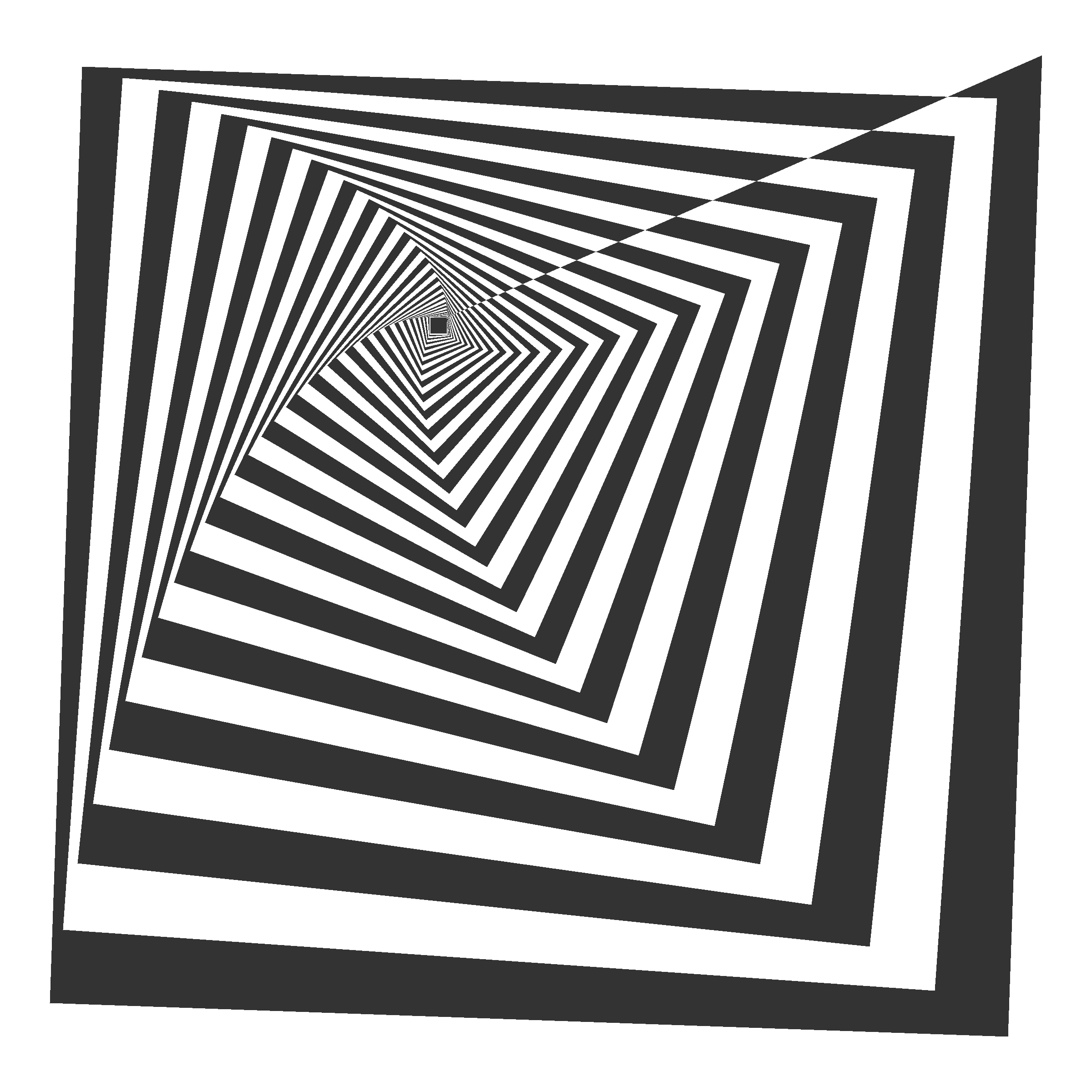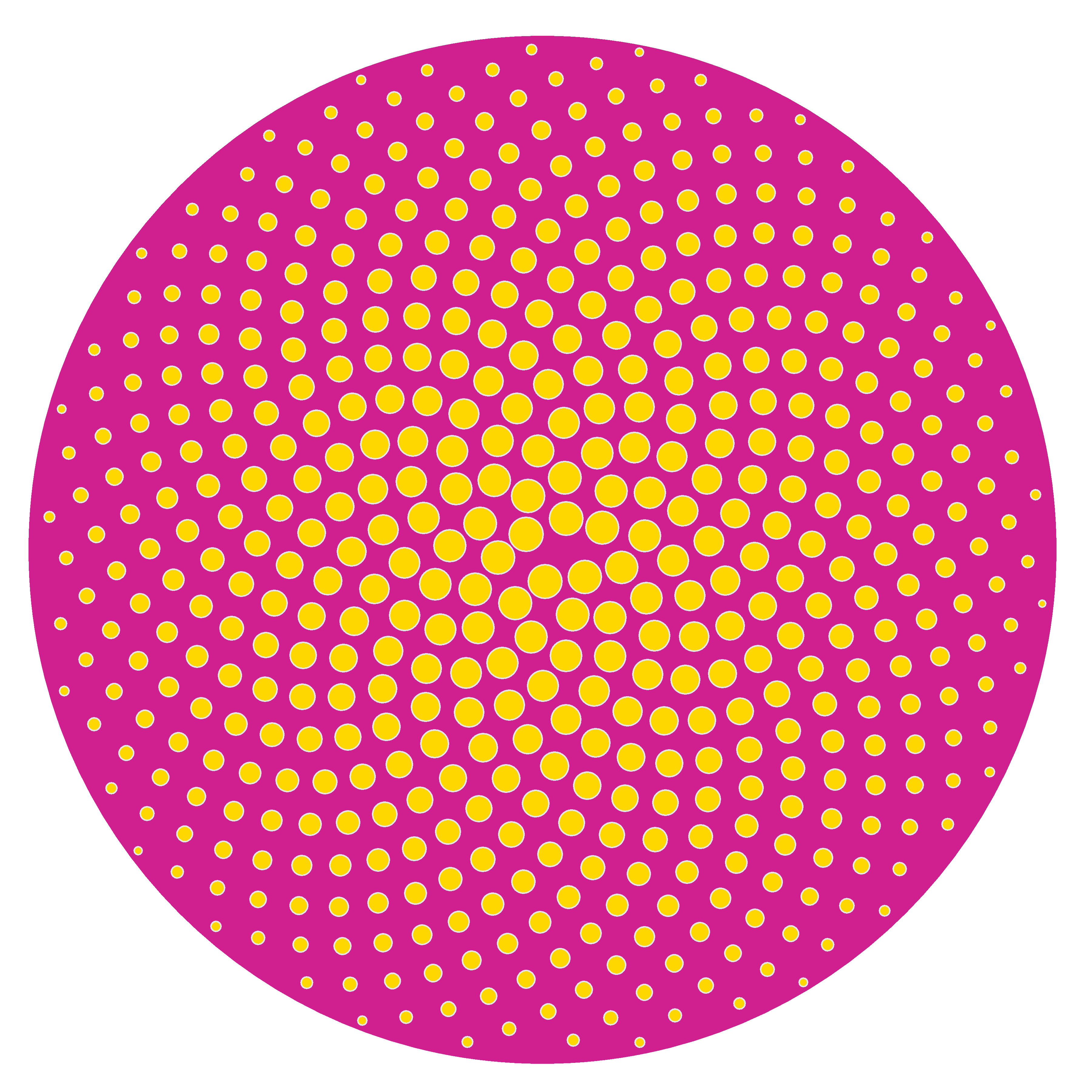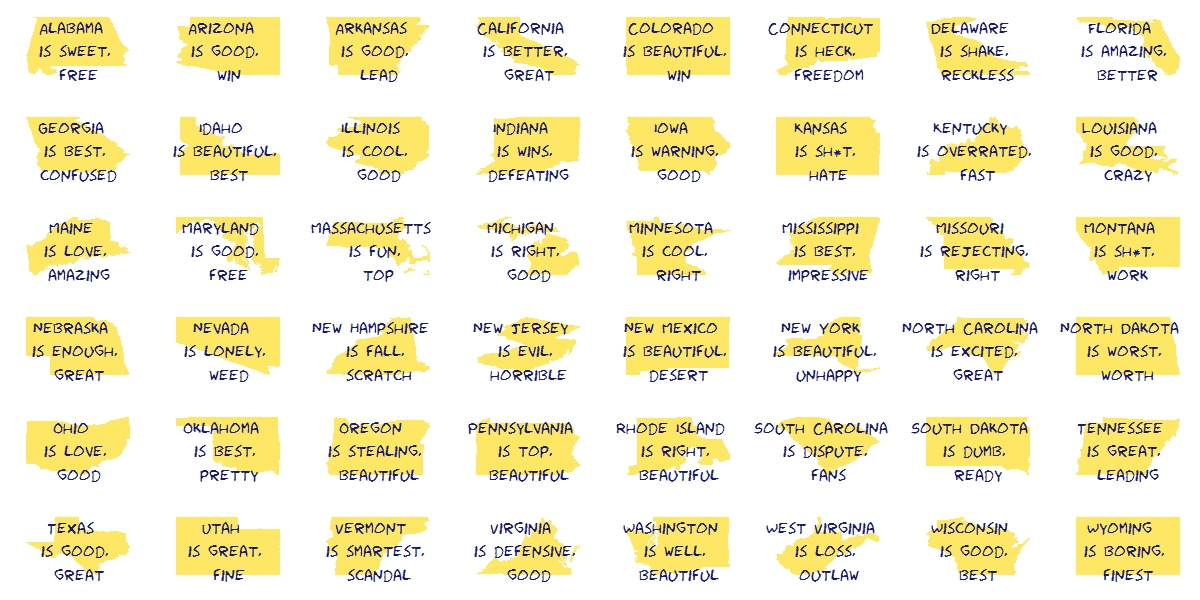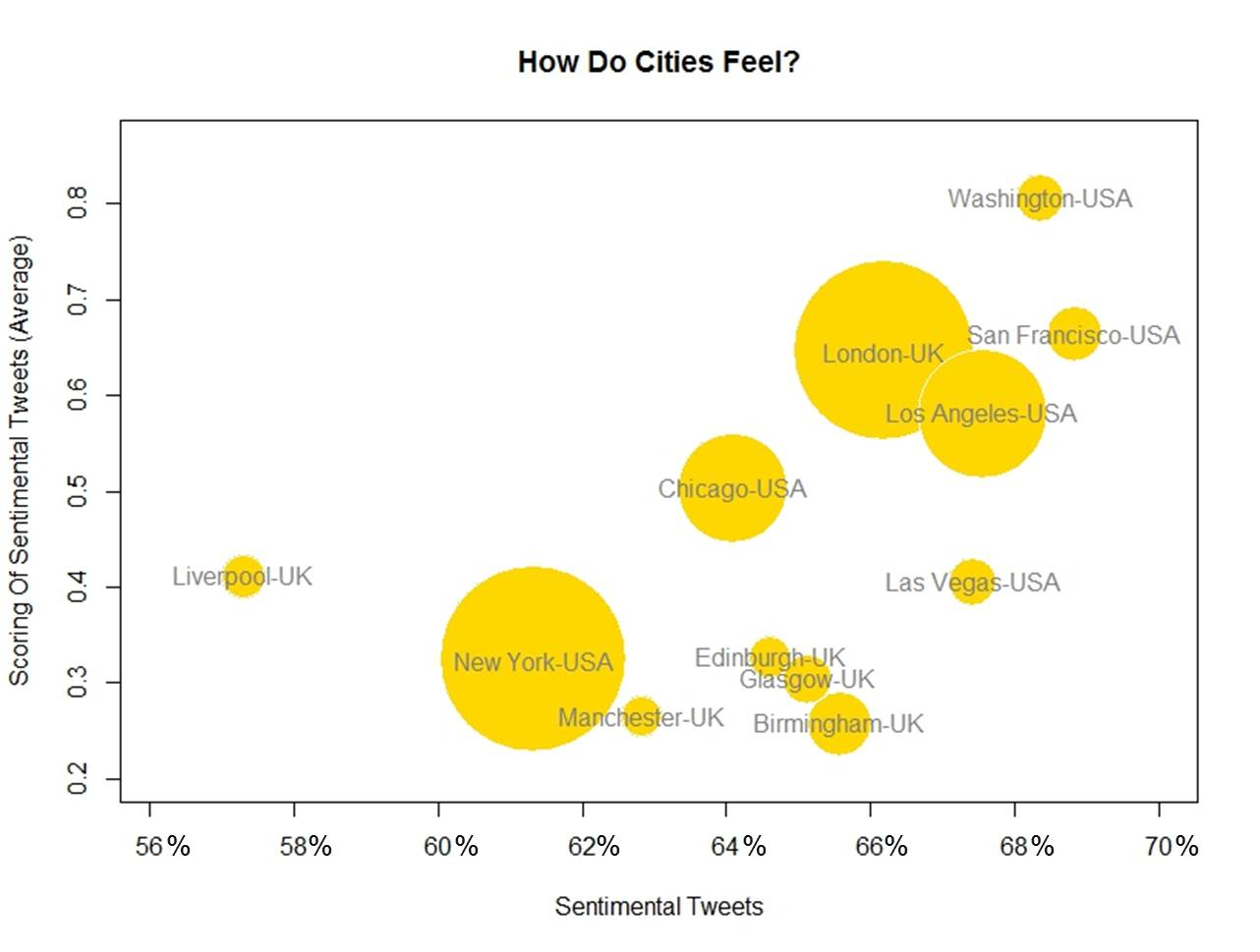# Tweetable Mathematical Art With R

Sin ese peso ya no hay gravedad
Sin gravedad ya no hay anzuelo
(Mira cómo vuelo, Miss Caffeina)

I love messing around with R to generate mathematical patterns. I always get surprised doing it and gives me lot of satisfaction. I also learn lot of things doing it: not only about R, but also about mathematics. It is one of my favourite hobbies. Some time ago, I published this post showing some drawings, each of them generated with less than 280 characters of code, to be shared on Twitter. This post came to appear in Hacker News, which provoked an incredible peak on visits to my blog. Some comments in the Hacker News entry are very interesting.

This Summer I delved into this concept of Tweetable Art publishing several drawings together with the R code to generate them. In this post I will show some.

Vertiginous Spiral

I came up with this image inspired by this nice pattern. It is a turtle graphic inspired pattern but instead of drawing lines I use geom_polygon to colour the resulting image in black and white:Code:

```library(tidyverse)
df <- data.frame(x=0, y=0)
for (i in 2:500){
df[i,1] <- df[i-1,1]+((0.98)^i)*cos(i)
df[i,2] <- df[i-1,2]+((0.98)^i)*sin(i)
}
ggplot(df, aes(x,y)) +
geom_polygon()+
theme_void()
```

Slight modifications of the code can generate appealing patterns like this:Marine Creature

A combination of sines and cosines. It reminds me a jellyfish:Code:

```library(tidyverse)
seq(from=-10, to=10, by = 0.05) %>%
expand.grid(x=., y=.) %>%
ggplot(aes(x=(x^2+pi*cos(y)^2), y=(y+pi*sin(x)))) +
geom_point(alpha=.1, shape=20, size=1, color="black")+
theme_void()+coord_fixed()
```

Summoning Cthulhu

The name is inspired in an answer from Mara Averick to this tweet. It is a modification of the marine creature in polar coordinates:Code:

```library(tidyverse)
seq(-3,3,by=.01) %>%
expand.grid(x=., y=.) %>%
ggplot(aes(x=(x^3-sin(y^2)), y=(y^3-cos(x^2)))) +
geom_point(alpha=.1, shape=20, size=0, color="white")+
theme_void()+
coord_fixed()+
theme(panel.background = element_rect(fill="black"))+
coord_polar()
```

Naive Sunflower

Sunflowers arrange their seeds according a mathematical pattern called phyllotaxis, whic inspires this image. If you want to create your own flowers, you can do this Datacamp’s project. It’s free and will introduce you to the amazing world of `ggplot2`, my favourite package to create images:Code:

```library(ggplot2)
a=pi*(3-sqrt(5))
n=500
ggplot(data.frame(r=sqrt(1:n),t=(1:n)*a),
aes(x=r*cos(t),y=r*sin(t)))+
geom_point(aes(x=0,y=0),
size=190,
colour="violetred")+
geom_point(aes(size=(n-r)),
shape=21,fill="gold",
colour="gray90")+
theme_void()+theme(legend.position="none")
```

Silk Knitting

It is inspired by this other pattern. A lot of almost transparent white points ondulating according to sines and cosines on a dark coloured background:Code:

```library(tidyverse)
seq(-10, 10, by = .05) %>%
expand.grid(x=., y=.) %>%
ggplot(aes(x=(x+sin(y)), y=(y+cos(x)))) +
geom_point(alpha=.1, shape=20, size=0, color="white")+
theme_void()+
coord_fixed()+
theme(panel.background = element_rect(fill="violetred4"))
```

Try to modify them and generate your own patterns: it is a very funny way to learn R.

Note: in order to make them better readable, some of the pieces of code below may have more than 280 characters but removing unnecessary characters (blanks or carriage return) you can reduce them to make them tweetable.

# A Shiny App to Create Sentimental Tweets Based on Project Gutenberg Books

There was something about them that made me uneasy, some longing and at the same time some deadly fear – Dracula (Stoker, Bram)

Twitter is a very good source of inspiration. Some days ago I came across with this:

The tweet refers to a presentation (in Spanish) available here, which is a very concise and well illustrated document about the state-of-the-art of text mining in R. I discovered there several libraries that I will try to use in the future. In this experiment I have used one of them: the `syuzhet` package. As can be read in the documentation:

this package extracts sentiment and sentiment-derived plot arcs from text using three sentiment dictionaries conveniently packaged for consumption by R users. Implemented dictionaries include `syuzhet` (default) developed in the Nebraska Literary Lab, `afinn` developed by Finn Arup Nielsen, `bing` developed by Minqing Hu and Bing Liu, and `nrc` developed by Mohammad, Saif M. and Turney, Peter D.

You can find a complete explanation of the package in its vignette. A very interesting application of these techniques is the Sentiment Graph of a book, which represents how sentiment changes over time. This is the Sentiment Graph of Romeo and Juliet, by William Shakespeare, taken from Project Alexandria:Darkest sentiments can be seen at the end of the book, where the tragedy reaches its highest level. It is also nice to see how sentiments are cyclical. This graphs can be very useful for people who just want to read happy endings books (my sister is one of those).

Inspired by this analysis, I have done another experiment in which I download a book from Project Gutenberg and measure sentiment of all its sentences. Based on this measurement, I filter top 5% (positive or negative sentiment) sentences to build tweets. I have done a Shiny app where all these steps are explained. The app is available here.

From a technical point of view I used `selectize` JavaScript library to filter books in a flexible way. I customized as well the appearance with CSS bootstrap from Bootswatch as explained here.

This is the code of the experiment.

`UI.R`:

```library(shiny)

fluidPage(theme = "bootstrap.css",

titlePanel(h1("Sentimental Tweets from Project Gutenberg Books", align="center"),
windowTitle="Tweets from Project Gutenberg"),
sidebarLayout(
sidebarPanel(

selectInput(
'book', 'Choose a book:',
multiple=FALSE,
selectize = TRUE,
choices=c("Enter some words of title or author" = "", gutenberg_works\$searchstr)
),

label = "Choose sentiment:",
choices = c("Dark"="1", "Bright"="20"),
selected="1",
inline=TRUE),

label = "Choose a method to measure sentiment:",
choices = c("syuzhet", "bing", "afinn", "nrc"),
selected="syuzhet",
inline=TRUE),

label = "Number of characters (max):",
choices = list("140", "280"),
inline=TRUE),

checkboxInput(inputId = "auth",
label = "Add author",
value=FALSE),

checkboxInput(inputId = "titl",
label = "Add title",
value=FALSE),

checkboxInput(inputId = "post",
value=TRUE),

label="Something else?",
placeholder="Maybe a #hastag?"),

actionButton('do','Go!',
class="btn btn-success action-button",
css.class="btn btn-success")
),

mainPanel(
tags\$br(),
p("First of all, choose a book entering some keywords of its
title or author and doing dropdown navigation. Books are
downloaded from Project Gutenberg. You can browse the complete
catalog", tags\$a(href = "https://www.gutenberg.org/catalog/", "here.")),

p("After that, choose the sentiment of tweets you want to generate.
There are four possible methods than can return slightly different results.
All of them assess the sentiment of each word of a sentence and sum up the
result to give a scoring for it. The more negative is this scoring,
the", em("darker") ,"is the sentiment. The more positive, the ", em("brighter."),
" You can find a nice explanation of these techniques",
tags\$a(href = "http://www.matthewjockers.net/2017/01/12/resurrecting/", "here.")),

p("Next parameters are easy: you can add the title and author of the book where
sentence is extracted as well as a link to my blog and any other string you want.
Clicking on the lower button you will get after some seconds a tweet below.
Click as many times you want until you like the result."),

p("Finally, copy, paste and tweet. ",strong("Enjoy it!")),
tags\$br(),
tags\$blockquote(textOutput("tweet1")),
tags\$br()

)))
```

`Server.R`:

```library(shiny)

function(input, output) {

values <- reactiveValues(default = 0)

observeEvent(input\$do,{
values\$default <- 1
})

book <- eventReactive(input\$do, {
GetTweet(input\$book, input\$meth, input\$sent, input\$char,
input\$auth, input\$titl, input\$post, input\$adds)
})

output\$tweet1 <- renderText({
if(values\$default == 0){
"Your tweet will appear here ..."
}
else{
book()
}
})
}
```

`Global.R`:

```library(gutenbergr)
library(dplyr)
library(stringr)
library(syuzhet)

x <- tempdir() # Read the Project Gutenberg catalog and filter english works. I also create a column with # title and author to make searchings gutenberg_metadata %>%
filter(has_text, language=="en", gutenberg_id>0, !is.na(author)) %>%
mutate(searchstr=ifelse(is.na(author), title, paste(title, author, sep= " - "))) %>%
mutate(searchstr=str_replace_all(searchstr, "[\r\n]" , "")) %>%
group_by(searchstr) %>%
summarize(gutenberg_id=min(gutenberg_id)) %>%
ungroup() %>%
na.omit() %>%
filter(str_length(searchstr)<100)-> gutenberg_works

# This function generates a tweet according the UI settings (book, method, sentiment and
# number of characters). It also appends some optional strings at the end
GetTweet = function (string, method, sentim, characters,
author, title, link, hastag)
{
# Obtain gutenberg_id from book
gutenberg_works %>%
filter(searchstr == string) %>%
select(gutenberg_id) %>% .\$gutenberg_id -> result

# Download text, divide into sentences and score sentiment. Save results to do it once and
# optimize performance
if(!file.exists(paste0(x,"/","book",result,"_",method,".RDS")))
{
book[,2] %>%
as.data.frame() %>%
.\$text %>%
paste(collapse=" ") -> text

sentences_v <- get_sentences(text)
sentiment_v <- get_sentiment(sentences_v, method=method) data.frame(sentence=sentences_v, sentiment=sentiment_v) %>%
mutate(length=str_length(sentence)) -> results
saveRDS(results, paste0(x,"/","book",result,"_",method,".RDS"))
}

# Paste optional strings to append at the end
post=""
if (title)  post=paste("-", book_info[,"title"], post, sep=" ")
if (author) post=paste0(post, " (", str_trim(book_info[,"author"]), ")")
if (link)   post=paste(post, "https://wp.me/p7VZWY-16S", sep=" ")
post=paste(post, hastag, sep=" ")
length_post=nchar(post)

# Calculate 5% quantiles
results %>%
filter(length<=(as.numeric(characters)-length_post)) %>%
mutate(sentiment=jitter(sentiment)) %>%
mutate(group = cut(sentiment,
include.lowest = FALSE,
labels = FALSE,
breaks = quantile(sentiment, probs = seq(0, 1, 0.05)))) -> results

# Obtain a sample sentence according sentiment and append optional string to create tweet
results %>%
filter(group==as.numeric(sentim)) %>%
sample_n(1) %>%
select(sentence) %>%
.\$sentence %>%
as.character() %>%
str_replace_all("[.]", "") %>%
paste(post, sep=" ") -> tweet

return(tweet)

}
```

# Silhouettes

Romeo, Juliet, balcony in silhouette, makin o’s with her cigarette, it’s juliet (Flapper Girl, The Lumineers)

Two weeks ago I published this post for which designed two different visualizations. At the end, I decided to place words on the map of the United States. The discarded visualization was this other one, where I place the words over the silhouette of each state:I do not want to set aside this chart because I really like it and also because I think it is a nice example of the possibilities one have working with R.

Here you have the code. It substitutes the fragment of the code headed by “Visualization” of the original post:

```library(ggplot2)
library(maps)
library(gridExtra)
library(extrafont)
opt=theme(legend.position="none",
panel.background = element_blank(),
panel.grid = element_blank(),
axis.ticks=element_blank(),
axis.title=element_blank(),
axis.text =element_blank(),
plot.title = element_text(size = 28))
vplayout=function(x, y) viewport(layout.pos.row = x, layout.pos.col = y)
grid.newpage()
jpeg(filename = "States In Two Words.jpeg", width = 1200, height = 600, quality = 100)
pushViewport(viewport(layout = grid.layout(6, 8)))
for (i in 1:nrow(table))
{
wd=subset(words, State==as.character(table\$"State name"[i]))
p=ggplot() + geom_polygon( data=subset(map_data("state"), region==tolower(table\$"State name"[i])), aes(x=long, y=lat, group = group), colour="white", fill="gold", alpha=0.6, linetype=0 )+opt
print(p, vp = vplayout(floor((i-1)/8)+1, i%%8+(i%%8==0)*8))
txt=paste(as.character(table\$"State name"[i]),"\n is", wd\$word1,"\n and", wd\$word2, sep=" ")
grid.text(txt, gp=gpar(font=1, fontsize=16, col="midnightblue", fontfamily="Humor Sans"), vp = viewport(layout.pos.row = floor((i-1)/8)+1, layout.pos.col = i%%8+(i%%8==0)*8))
}
dev.off()
```

# The United States In Two Words

Sweet home Alabama, Where the skies are so blue; Sweet home Alabama, Lord, I’m coming home to you (Sweet home Alabama, Lynyrd Skynyrd)

This is the second post I write to show the abilities of `twitteR` package and also the second post I write for KDnuggets. In this case my goal is to have an insight of what people tweet about american states. To do this, I look for tweets containing the exact phrase “[STATE NAME] is” for every states. Once I have the set of tweets for each state I do some simple text mining: cleaning, standardizing, removing empty words and crossing with these sentiment lexicons. Then I choose the two most common words to describe each state. You can read the original post here. This is the visualization I produced to show the result of the algorithm:Since the right side of the map is a little bit messy, in the original post you can see a table with the couple of words describing each state. This is just an experiment to show how to use and combine some interesting tools of R. If you don’t like what Twitter says about your state, don’t take it too seriously.

This is the code I wrote for this experiment:

```# Do this if you have not registered your R app in Twitter
library(RCurl)
setwd("YOUR-WORKING-DIRECTORY-HERE")
if (!file.exists('cacert.perm'))
{
}
consumerKey = "YOUR-CONSUMER_KEY-HERE"
consumerSecret = "YOUR-CONSUMER-SECRET-HERE"
Cred <- OAuthFactory\$new(consumerKey=consumerKey,
consumerSecret=consumerSecret,
requestURL=requestURL,
accessURL=accessURL,
authURL=authURL)
Cred\$handshake(cainfo=system.file("CurlSSL", "cacert.pem", package="RCurl"))
# Start here if you have already your twitter authentification.Rdata file
library(RCurl)
library(XML)
options(RCurlOptions = list(cainfo = system.file("CurlSSL", "cacert.pem", package = "RCurl")))
#Read state names from wikipedia
webpage=getURL("http://simple.wikipedia.org/wiki/List_of_U.S._states")
table=table[!(table\$"State name" %in% c("Alaska", "Hawaii")), ]
#Extract tweets for each state
results=data.frame()
for (i in 1:nrow(table))
{
tweets=searchTwitter(searchString=paste("'\"", table\$"State name"[i], " is\"'",sep=""), n=200, lang="en")
tweets.df=twListToDF(tweets)
results=rbind(cbind(table\$"State name"[i], tweets.df), results)
}
results=results[,c(1,2)]
colnames(results)=c("State", "Text")
library(tm)
#Lexicons
pos = scan('positive-words.txt',  what='character', comment.char=';')
neg = scan('negative-words.txt',  what='character', comment.char=';')
posneg=c(pos,neg)
results\$Text=tolower(results\$Text)
results\$Text=gsub("[[:punct:]]", " ", results\$Text)
# Extract most important words for each state
words=data.frame(Abbreviation=character(0), State=character(0), word1=character(0), word2=character(0), word3=character(0), word4=character(0))
for (i in 1:nrow(table))
{
doc=subset(results, State==as.character(table\$"State name"[i]))
doc.vec=VectorSource(doc[,2])
doc.corpus=Corpus(doc.vec)
stopwords=c(stopwords("english"), tolower(unlist(strsplit(as.character(table\$"State name"), " "))), "like")
doc.corpus=tm_map(doc.corpus, removeWords, stopwords)
TDM=TermDocumentMatrix(doc.corpus)
TDM=TDM[Reduce(intersect, list(rownames(TDM),posneg)),]
v=sort(rowSums(as.matrix(TDM)), decreasing=TRUE)
words=rbind(words, data.frame(Abbreviation=as.character(table\$"Abbreviation"[i]), State=as.character(table\$"State name"[i]),
}
# Visualization
require("sqldf")
statecoords=as.data.frame(cbind(x=state.center\$x, y=state.center\$y, abb=state.abb))
#To make names of right side readable
texts=sqldf("SELECT a.abb,
CASE WHEN a.abb IN ('DE', 'NJ', 'RI', 'NH') THEN a.x+1.7
WHEN a.abb IN ('CT', 'MA') THEN a.x-0.5  ELSE a.x END as x,
CASE WHEN a.abb IN ('CT', 'VA', 'NY') THEN a.y-0.4 ELSE a.y END as y,
b.word1, b.word2 FROM statecoords a INNER JOIN words b ON a.abb=b.Abbreviation")
texts\$col=rgb(sample(0:150, nrow(texts)),sample(0:150, nrow(texts)),sample(0:150, nrow(texts)),max=255)
library(maps)
jpeg(filename = "States In Two Words v2.jpeg", width = 1200, height = 600, quality = 100)
map("state", interior = FALSE, col="gray40", fill=FALSE)
map("state", boundary = FALSE, col="gray", add = TRUE)
text(x=as.numeric(as.character(texts\$x)), y=as.numeric(as.character(texts\$y)), apply(texts[,4:5] , 1 , paste , collapse = "\n" ), cex=1, family="Humor Sans", col=texts\$col)
dev.off()
```

# How Do Cities Feel?

If you are lost and feel alone, circumnavigate the globe (For You, Coldplay)

You can not consider yourself a R-blogger until you do an analysis of Twitter using `twitteR `package. Everybody knows it. So here I go.

Inspired by the fabulous work of Jonathan Harris I decided to compare human emotions of people living (or twittering in this case) in different cities. My plan was analysing tweets generated in different locations of USA and UK with one thing in common: all of them must contain the string “I FEEL”. These are the main steps I followed:

• Locate cities I want to analyze using world cities database of `maps` package
• Download tweets around these locations using `searchTwitter` function of `twitteR` package.
• Cross tweets with positive and negative lists of words and calculate a simple scoring for each tweet as number of positive words – number of negative words
• Calculate how many tweets have non-zero scoring; since these tweets put into words some emotion I call them sentimental tweets
• Represent cities in a bubble chart where x-axis is percentage of sentimental tweets, y-axis is average scoring and size of bubble is population

This is the result of my experiment:These are my conclusions (please, do not take it seriously):

• USA cities seem to have better vibrations and are more sentimental than UK ones
• Capital city is the happiest one for both countries
• San Francisco (USA) is the most sentimental city of the analysis; on the other hand, Liverpool (UK) is the coldest one
• The more sentimental, the better vibrations

From my point of view, this analysis has some important limitations:

• It strongly depends on particular events (i.e. local football team wins the championship)
• I have no idea of what kind of people is behind tweets
• According to my experience, `searchTwitter `only works well for a small number of searches (no more than 300); for larger number of tweets to return, it use to give malformed JSON response error from server

Anyway, I hope it will serve as starting point of some other analysis in the future. At least, I learned interesting things about R doing it.

Here you have the code:

```library(twitteR)
library(RCurl)
library(maps)
library(plyr)
library(stringr)
library(bitops)
library(scales)
#Register
if (!file.exists('cacert.perm'))
{
}
consumerKey = "YOUR CONSUMER KEY HERE"
consumerSecret = "YOUR CONSUMER SECRET HERE"
Cred <- OAuthFactory\$new(consumerKey=consumerKey,
consumerSecret=consumerSecret,
requestURL=requestURL,
accessURL=accessURL,
authURL=authURL)
Cred\$handshake(cainfo=system.file("CurlSSL", "cacert.pem", package="RCurl"))
#Save credentials
options(RCurlOptions = list(cainfo = system.file("CurlSSL", "cacert.pem", package = "RCurl")))
#Cities to analyze
cities=data.frame(
CITY=c('Edinburgh', 'London', 'Glasgow', 'Birmingham', 'Liverpool', 'Manchester',
'New York', 'Washington', 'Las Vegas', 'San Francisco', 'Chicago','Los Angeles'),
COUNTRY=c("UK", "UK", "UK", "UK", "UK", "UK", "USA", "USA", "USA", "USA", "USA", "USA"))
data(world.cities)
cities2=world.cities[which(!is.na(match(
str_trim(paste(world.cities\$name, world.cities\$country.etc, sep=",")),
str_trim(paste(cities\$CITY, cities\$COUNTRY, sep=","))
))),]
cities2\$SEARCH=paste(cities2\$lat, cities2\$long, "10mi", sep = ",")
cities2\$CITY=cities2\$name
tweets=data.frame()
for (i in 1:nrow(cities2))
{
tw=searchTwitter("I FEEL", n=400, geocode=cities2[i,]\$SEARCH)
tweets=rbind(merge(cities[i,], twListToDF(tw),all=TRUE), tweets)
}
#Save tweets
write.csv(tweets, file="tweets.csv", row.names=FALSE)
#Import csv file
hu.liu.pos = scan('lexicon/positive-words.txt',  what='character', comment.char=';')
hu.liu.neg = scan('lexicon/negative-words.txt',  what='character', comment.char=';')
#Function to clean and score tweets
score.sentiment=function(sentences, pos.words, neg.words, .progress='none')
{
require(plyr)
require(stringr)
scores=laply(sentences, function(sentence, pos.word, neg.words) {
sentence=gsub('[[:punct:]]','',sentence)
sentence=gsub('[[:cntrl:]]','',sentence)
sentence=gsub('\\d+','',sentence)
sentence=tolower(sentence)
word.list=str_split(sentence, '\\s+')
words=unlist(word.list)
pos.matches=match(words, pos.words)
neg.matches=match(words, neg.words)
pos.matches=!is.na(pos.matches)
neg.matches=!is.na(neg.matches)
score=sum(pos.matches) - sum(neg.matches)
return(score)
}, pos.words, neg.words, .progress=.progress)
scores.df=data.frame(score=scores, text=sentences)
return(scores.df)
}
cities.scores=score.sentiment(city.tweets[1:nrow(city.tweets),], hu.liu.pos, hu.liu.neg, .progress='text')
cities.scores\$pos2=apply(cities.scores, 1, function(x) regexpr(",",x)-1)
cities.scores\$CITY=apply(cities.scores, 1, function(x) substr(x, 1, x))
cities.scores=merge(x=cities.scores, y=cities, by='CITY')
df1=aggregate(cities.scores["score"], by=cities.scores[c("CITY")], FUN=length)
names(df1)=c("CITY", "TWEETS")
cities.scores2=cities.scores[abs(cities.scores\$score)>0,]
df2=aggregate(cities.scores2["score"], by=cities.scores2[c("CITY")], FUN=length)
names(df2)=c("CITY", "TWEETS.SENT")
df3=aggregate(cities.scores2["score"], by=cities.scores2[c("CITY")], FUN=mean)
names(df3)=c("CITY", "TWEETS.SENT.SCORING")
#Data frame with results
df.result=join_all(list(df1,df2,df3,cities2), by = 'CITY', type='full')
#Plot results